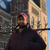# App Development

Discussion board where members can learn more about Qlik Sense App Development and Usage.

Announcements
April 22, 2PM EST: Learn about GeoOperations in Qlik Sense SaaS READ MORE
cancel
Showing results for
Search instead for
Did you mean:Creator

## Sum of Expression values

Hello Guys , i want to add below expression and create new column as Summation. Kindly let me know how to do ?

("AverageHrs"/30)*0.8)

("AverageHrs"/30)

(("AverageHrs"/30)*1.2))

I want to add values of all three expression and assign it new field as Summation

I tried like this but its not working

Sum((("Average Flow3Hrs"/30)*0.8)and ("Average Flow3Hrs"/30) and(("Average Flow3Hrs"/30)*1.2))

as summation,

Thanks,

ravi

19 RepliesChampion

Hi,

if you want to sum on the script, you should use Group by, example:

Table:

load

field1,

field2,

"Average Flow3Hrs",

Sum((("Average Flow3Hrs"/30)*0.8)+("Average Flow3Hrs"/30) +(("Average Flow3Hrs"/30)*1.2))

as Summation

from...

Group by

field1,

field2,

"Average Flow3Hrs";MVP

are you may be looking for this

(("Average Flow3Hrs"/30)*0.8) + ("Average Flow3Hrs"/30) + ("Average Flow3Hrs"/30)*1.2))

as summation,Creator
Author

Thanks YoussefCreator
Author

Thanks sunny , I am still working on it . shall keep postedCreator
Author

hey Sunny

Kindly see my expression it does not give me desired result .

((("Flow3 Hrs per")/(("Average Flow3Hrs"/30)*0.8))+

(("Flow3 Hrs per")/ (("Average Flow3Hrs"/30)))

+ (("Flow3 Hrs per")/(("Average Flow3Hrs"/30)*1.2))) as impactfor3

I am not able to locate impactfor3

Thanks,

RaviMVP

What does it give and what did you hope it would giveCreator
Author

Hello Sunny ,

please find details below

Flow3 Hrs per" has a value of 50 , 500, 400

Average Flow3Hrs has value of 154

((("50")/(("Average Flow3Hrs"/30)*0.8))+

(("500")/ (("Average Flow3Hrs"/30)))

+ (("400")/(("Average Flow3Hrs"/30)*1.2))) as impactfor3

impactfor3 should give me addition of all these 3 expression .MVP

What is your complete script here? It seems you need some kind of Aggregation here in a resident load to calculate the Average and then left join into your main table. and then finally you will need to use a resident load to do this calculationCreator
Author

[Project1]:

LOAD

"Product Line",

"Flow3 Hrs per",

"Average Flow3Hrs",

(("Average Flow3Hrs"/30)*0.8) + ("Average Flow3Hrs"/30) + ((("Average Flow3Hrs"/30)*1.2))

as flow3summation,

(("Flow3 Hrs per")/(("Average Flow3Hrs"/30)*0.8))+(("Flow3 Hrs per")/(("Average Flow3Hrs"/30)))+(("Flow3 Hrs per")/(("Average Flow3Hrs"/30)*1.2)) as impactfor3

FROM [lib://AttachedFiles/Complexity_Mix.xlsx]

(ooxml, embedded labels, header is 1 lines, table is Model)

where "Product Line"='Terminals';

left Join ([Project1])

[Duration]:

Load

"Product Line",

"Average Flow3Hrs",

"Flow3 Hrs per",

((("Flow3 Hrs per")/(("Average Flow3Hrs"/30)*0.8))+

(("Flow3 Hrs per")/ (("Average Flow3Hrs"/30)))

+ (("Flow3 Hrs per")/(("Average Flow3Hrs"/30)*1.2))) as impactfor3

Resident [Project1]

Group by "Product Line";